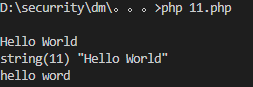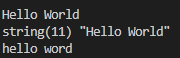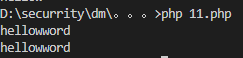php基础# 语法

<html>
<title>Example</title>
<body>
<?php
echo "Hi, I'm a PHP script!";//可以看到php语句在HTML中
?>
</body>
</html>

// 注释当前一行
/包裹内容全部注释/

## 输入输出语句

### 输出

echo “hellow word\n”;

var_dump(“hello word”);

printf(“%s”,“hellow word\n”);

var_export

<?php
echo "Hello World\n";
var_dump("Hello World");
printf("%s","hello word\n");
?>### 输入

fgets(STDIN): 获取输入的当前行
fgetc(STDIN)：获取一个字符
sscanf(string $str ,string KaTeX parse error: Expected ‘EOF’, got ‘&’ at position 17: …ormat [, mixed &̲… ])：将 str 按照 fromat 进行格式化解析 <?php$str = fgets(STDIN);//获取当前行
sscanf($str,"%s %d",$s,$d); echo$s,$d; ?>## 变量 $a=1;
$b; %c="hellow word";$+变量名

### 变量作用域

$_SERVER：//服务器和执行环境信息，是一个包含了诸如头信息(header)、路径(path)、以及脚本位置(script locations)等等信息的数组$_GET：HTTP GET //变量，通过URL参数传递给当前脚本的变量的数组。 自动url解码
$_POST：HTTP POST// 变量，HTTP POST请求的。Content-Type 是 application/x-www-formurlencoded 或 multipart/form-data 时，会将变量以关联数组形式传入当前脚本$_FILES：HTTP //文件上传变量，通过 HTTP POST方式上传到当前脚本的项目的数组
$_REQUEST：HTTP //Request 变量，默认情况下包含了$_GET(自动url解码)，$_POST 和$_COOKIE 的数组。
$_SESSION：//Session 变量，当前脚本可用 SESSION 变量的数组$_ENV：//环境变量，通过环境方式传递给当前脚本的变量的数组。
$_COOKIE：//HTTP Cookies，通过 HTTP Cookies方式传递给当前脚本的变量的数组$php_errormsg：//前一个错误信息
$HTTP_RAW_POST_DATA：//原生POST数据$http_response_header：//HTTP 响应头，使用HTTP 包装器时，$http_response_header 将会被HTTP 响应头信息填充。$argc：//传递给脚本的参数数目，传递给脚本的参数数目
$argv：传递给脚本的参数数组，传递给脚本的参数数组 ## 数据类型 ### 数字型 $int1=1234 //十进制正整数
$int2=-1234 //负整数$int3=01234 //八进制整数
$int4=0x1234 //十六进制整数$float1=666.66 //浮点数
$float2=6.6666e2 //表示6.6666乘以10的2次方，为指数形式的浮点数 ### 字符串型 $c1 = 'c'
$c2 = "c"$s1 = 'str'
$s2 = "str" ### 布尔型 只有true和false $a = true;
$b = false; ### 数组型 #### 定义 PHP中的数组实际上是一个有序映射。映射是一种把 values 关联到 keys 的类型。 key的值必须是整型或者字符串型，values值可以是任何类型。 定义数组时如果不加key键，key值默认从0开始（下标从0）。 $array1 = [
"foo" => "bar",
"bar" => "foo",
];
$array2 = ['a','b']; #等价于$array2 = [ 0 => "a", 1 => "b"];

& 变量地址
@ 屏蔽错误信息（扔到函数前头）
-> 应用对象方法或者属性
=> 用于数组元素赋值）
?: 三目运算符

## 流程控制语句

### if-elseif-else

if ($a >$b) {
echo "a is bigger than b";
} elseif ($a ==$b) {
echo "a is equal to b";
} else {
echo "a is smaller than b";
}

switch ($i) { case 0: echo "i equals 0"; break; case 1: echo "i equals 1"; break; case 2: echo "i equals 2"; break; } ### while $i = 1;
while ($i <= 10) { echo$i++;
}

### do-while

$i = 0; do { echo$i;
echo $i; } ### foreach $arr = array("one", "two", "three");
foreach ($arr as$key => $value) { echo "Key:$key; Value: $value<br />\n"; } foreach ($arr as $value) { echo "Value:$value<br />\n";
}

## 函数

<?php
function add_some_str_a($string) {$string .= 'and something extra.';
}
function add_some_str_b(&$string) {$string .= 'and something extra.';
}
$str = 'This is a string, '; add_some_str_a($str);
echo $str."\n"; add_some_str_b($str);
echo \$str."\n";
?>